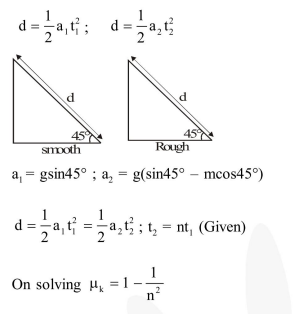# A smooth block is released at rest on a

Question:

A smooth block is released at rest on a $45^{\circ}$ incline and then slides a distance $\mathrm{d}$. The time taken to slide is $\mathrm{n}$ times as much to slide on rough incline than on a smooth incline. The coefficient of friction is-

1. $\mu_{k}=1-\frac{1}{n^{2}}$

2. $\mu_{k}=\sqrt{1-\frac{1}{n^{2}}}$

3. $\mu_{s}=1-\frac{1}{n^{2}}$

4. $\mu_{\mathrm{s}}=\sqrt{1-\frac{1}{\mathrm{n}^{2}}}$

Correct Option: 1

Solution: